This post aims to demystify bitwise operations in JavaScript, as they can be useful for a myriad of applications. They are also very useful, for example, when building emulators, an exercise I’d definitely recommend.

Even though I will use JavaScript throughout the whole article, these are the absolute essentials for working with bit manipulation and will definitely be useful in whatever language you end up using.

## Bits, Bytes, Words, and Base-X Systems

There are many ways of representing the same numbers.

We humans naturally use the decimal system as a way of representing numbers. This means that we can count up to 10 - 1 using a single place. Once we reach 10 in any of the places of our number representation, we carry one over to the next place. The number 22 is nothing but 10 units repeated twice plus 2 units.

In the same way that the system I described above uses ten as its base, we could have chosen to use any number as a base.

Hexadecimal numbers, for example, are nothing but numbers represented using 16 as a base. Every place can count up to 16 - 1. Once we reach 16 in any of the places of our number representation, we will carry one over to the next place.

The reason why you see A, B, C, D, E and F being used in hexadecimal numbers is that we have agreed that these would be the symbols we would use to represent numbers bigger than 10 using a single character. In hexadecimal numbers A is 10, B is 11, C is 12, D is 13, E is 14 and F is 15.

In the same way that you represent the number 15 in decimals, you could represent it in hexadecimals using only F. The number 33 in hexadecimals can be represented as 21 since the 2 in the second place represents 16 twice and the 1 in the first place represents just that: 1. This is why it is important to know which base you are using to deal with numbers otherwise, you could have thought 21 was a decimal number and therefore interpreted it wrong.Binary numbers are nothing but numbers represented on base-2 and they have the exact same properties of any other base-x systems. Every time a place in a binary number exceeds 2 - 1 it will then carry one over to the next place.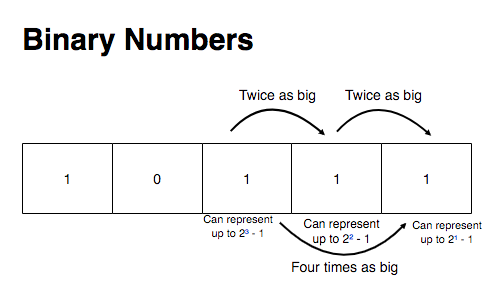If you have the binary number 1 and try to increment it by 1 it will then carry one over to the next place and the result will be 10, which represents 2. When you add 1 to that, since the first place is still smaller than 2, you will get 11, which represents 3. The binary number 100 is just another representation of 4.

The bigger your base, the more compact the representation. This means that representing a number using hexadecimals takes a lot less space than representing it in binary.

It’s important to know how to deal with binary numbers in computer science because that’s the way computers see numbers. In the end, it’s all zeros and ones.

Our computers are this huge pile of transistors, an electronic component which can only be on (1) or off (0) and therefore by grouping up transistors we can represent bigger numbers, but only in base 2.

A byte is a group of 8 bits, which means that we have 8 places to hold zeros or ones and therefore we can represent a total of 2⁸-1 different numbers.

If we want to be able to represent both positive and negative numbers in binary format, we set the leftmost bit to 1 for negative numbers and 0 for positive numbers.

To work with representations in different bases it is important to dissociate numbers from their representation and learn the general properties of those ways of representing numbers, and this is what I’m gonna do in this post.

## Representing Binaries, Octals, and Hexadecimals in JavaScript

Representing numbers in these different bases in JavaScript requires us to start that number literal with 0 followed by a letter.

• For hexadecimals, we start our representation with 0x
• For octals, we start our representation with 0o
• For binaries, we start our representation with 0b

This is part of the ES6 spec and can be found under the Numeric Literals section of the specification document.

It is also possible to represent numbers in other bases (up to 36) by passing an argument (the radix) when calling toString for a Number. For example:

(33).toString(2); // '100001'
(33).toString(8); // '41'
(33).toString(16); // '21'


In order to convert stringified representations in different bases to Number primitives, you can use parseInt, which takes a string and a radix.

parseInt('100001', 2); // 33
parseInt('41', 8); // 33
parseInt('21', 16); // 33


### Bitwise Operators

Before exploring how to perform common bit operations it is, of course, essential to know which operators are available for manipulating binary numbers and how they work.

It’s important to notice that whenever you use a bitwise operator, all of the operands get converted to signed 32-bit integers.

This means that 124, for example, instead of simply becoming 01111100, becomes 00000000 00000000 00000000 01111100.

It’s important to keep this in mind so that you know what happens if the representations you are using at first have a different number of bits. In this case, they will be padded with 0 and the signal will be kept (at the leftmost bit, as usual). This guarantees the binary numbers you are operating with will always have the same number of bits.

#### AND → &

Bitwise AND, when applied to a single binary digit, does exactly what you’d expect from AND: it returns 1 if both digits are 1.

When applied to two binary numbers it will return 1 whenever the corresponding bits of both are 1.

const foo = 0b10101; // 21
const bar = 0b10011; // 19

const result = foo & bar; // 17

result.toString(2); // '10001'


#### OR → |

Bitwise OR will return 1 whenever one of the corresponding bits of the operand is 1.

const foo = 0b10101; // 21
const bar = 0b10011; // 19

const result = foo | bar; // 23

result.toString(2); // '10111'


#### XOR → ^

Also known as exclusive or, it will return 1 whenever the two corresponding bits are different from each other.

This means that any combination of zeros and ones will result in 1, except 1 and 1, and 0 and 0.

const foo = 0b10101; // 21
const bar = 0b10011; // 19

const result = foo ^ bar; // 6

result.toString(2); // '110'


#### NOT → ~

NOT inverts an operand. Just as you’d expect, if it finds a 1 it turns it into a 0 and vice-versa. This transforms positive numbers into negative numbers and vice versa.

As you might remember, we use the leftmost bit of a binary number to indicate its signal.

Due to this, you may be lead to think that 0111, when negated would simply become 1111. That is not true.

The NOT operator is applied to each bit of a number individually. This means that 0111 would become 1000. This is also known as one’s complement.

#### Left Shift → <<

Left shift causes a binary representation, which goes into the left side of the operator, to be shifted by the number of bits specified in the right-side of the operator. It will add in 0s at the right when shifting.

const foo = 0b010101; // 21

const result = foo << 1; // 42

result.toString(2); // '101010'


When shifting to the left just imagine that you have an infinite number of zeros at the right, but no remaining space at the left so that when you shift a number to the left, the zero’s at the right get pulled in, but the leftmost number goes towards the wall.

Remember that operands are always converted to 32-bit integers. This causes only the 32nd bit (the leftmost bit) to be discarded when shifting.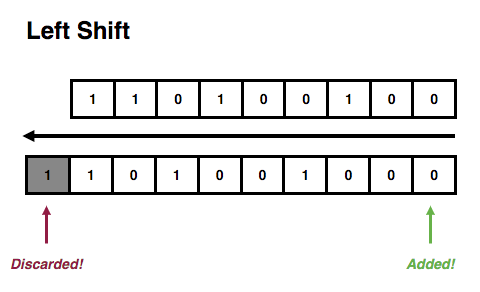#### Right Shift (Sign-Propagating) → >>

The right shift with sign-propagation, as the name implies, shifts a binary representation, which goes into the left side of the operator, to the right by the number of places specified in the right-side of the operator.

However, it is important to notice that the sign bit at the left will be added in from the left.

If a number’s leftmost bit is 1, when shifting with >>, we will add in 1’s to the left.

If a number’s leftmost bit is 0, we will add in 0’s to the left.

const leftmostOne = 0b11111111111111111111111111110000;
const leftmostZero = 0b01111111111111111111111111110000;

// This will fill-in 1's in the left
leftmostOne >> 2
// 0b11111111111111111111111111111100

// This will fill-in 0's in the left
leftmostZero >> 2
// 0b00011111111111111111111111111100


This is also known as an arithmetic right shift.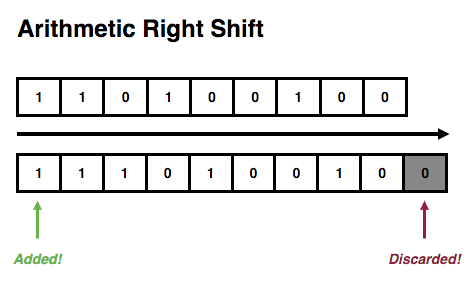#### Right Shift (Zero-Fill) → >>>

This right-shift also shifts a binary representation to the right, but always adds in 0s to the left, no matter what is the leftmost bit (sign-bit).

const leftmostOne = 0b11111111111111111111111111110000;
const leftmostZero = 0b01111111111111111111111111110000;

// This will fill-in 0's in the left
leftmostOne >>> 2
// 0b00111111111111111111111111111100

// This will also fill-in 0's in the left
leftmostZero >>> 2
// 0b00011111111111111111111111111100


This is also known as a logical right shift.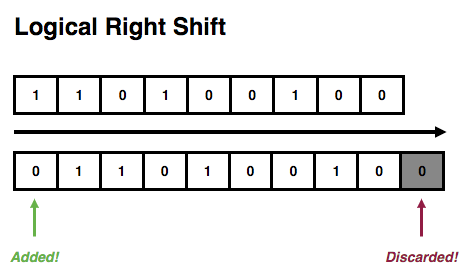## Addition, subtraction and Two’s Complement

Adding binary numbers follows the exact same procedure as adding decimal numbers.

As we have explained at the beginning of this post, when doing addition all we need to do is sum two numbers and if they exceed base - 1, which in the case of binary numbers is 1, we carry one over to the next place.

This is what happens when we add, for example, 19 (00010011) and 41 (00101001).

  00010011 → 19
+ 00101001 → 41
= 00111100 → 60


For subtraction, we just need to perform an addition between the first operand (minuend) and the inverse of the second operand (subtrahend).

However, for us to be able to do this, we need to know how to invert binary numbers. We do this using two’s complement.

Computerphile has this excellent video explaining why we use two’s complement and I don’t think I could explain these reasons in a better way. Instead, I will focus on briefly explaining what it is and how to get the two’s complement of a binary number.

We have already seen that obtaining one’s complement of a number consists of inverting all of its bits. Two’s complement consists of obtaining a number’s one’s complement and adding 1 to it.

Two’s complement allows us to obtain a number with an inverse signal.

Let’s say you have the number 3, represented in 8-bits as 00000011 and you want to get -3:

00000011 → Invert all bits (NOT)
11111101 → This is -3


Now, if you want to subtract 3 from 7 you can simply add 7 and -3:

  00000111 → 7
+ 11111101 → -3
00000100 → 4


Notice that if you have any carries that overflow the word’s length you can simply drop them as we’ve done in the case above.

Another way of thinking about two’s complement is imagining it as if the leftmost bit (the most significant bit) signed the corresponding value in that place as a negative value in such a way that if you had a 4-bit signed integer like 1011, that would mean that the bit for 8 is actually -8 since it has a 1 on it and then all the other bits are positive, resulting in -8 + 2 + 1, which is -4: the two’s complement of 4 (0100).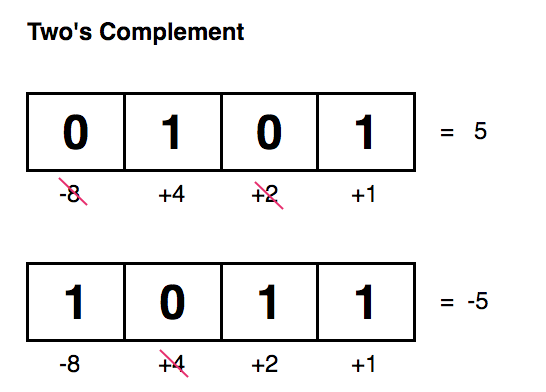const twoComplement = (num) => (~num + 0b1)


Knowing how to work with bitmasks is extremely important for operating efficiently with binary numbers.

A bitmask is simply a set of bits where the desired bits are 1s and the others are 0. We use these masks to perform operations against other binary numbers, as in the examples below.

### Getting a specific bit

Let’s say you had the number 10110101 and you wanted to get the third bit from right to left. Considering that the first bit is at index 0 we are gonna say that we want the bit at index 2.

1. Create a bitmask with 00000100. You can do this by shifting 1 by the index of the bit you want to get.

 00000001 << 2 = 00000100

2. Perform an AND operation.

     10110101
AND 00000100
00000100


Since bitwise operators deal with bits individually, this will change all bits except the third one. If the third bit was 0 it will remain as 0 and if it was 1 it will remain as 1, all other bits will become 0.

3. Now we can simply shift the result 2 places to the right, which will then put the unchanged bit in the first place of our binary number.

  00000100 >>> 2 → 00000001


#### Doing it in JavaScript

const getBit = (n, bitIndex) => {
const bitMask = 1 << bitIndex;
const result = n & bitMask;
return result >>> bitIndex;
}


### Setting a specific bit

It’s important here to make it very clear that what I mean by setting a specific bit means turning it to 1, and not just assigning any value to it.

In this example, I’m gonna set the third bit (index 2) in 10110001 to 1.

1. Let’s start by creating a bitmask with 1 in the position we want to set. We do this by shifting 1 to the left by the index of the bit we want to set.

 00000001 << 2 = 00000100

2. Now we can perform an OR so that all bits will remain the same, except the bit at the third position, which will then be set to 1.

     10110001
OR  00000100
10110101


#### Doing it in JavaScript

const setBit = (n, bitIndex) => {
const bitMask = 1 << bitIndex;
}


### Clearing a specific bit

Let’s say we want to clear an specific bit (setting it to 0).

In this example, we’re going to clear the third bit (index 2) in 10110101

1. We create a mask with 1 in the index we want to clear.

 00000001 << 2 = 00000100

2. We invert the mask using NOT.

 NOT 00000100 = 11111011

3. We perform an AND. This will make all the bits remain the same, except for the one in the same position as the 0 in the mask, since that will turn any bit to 0.

     10110101
AND 11111011
10110001


#### Doing it in JavaScript

const clearBit = (n, bitIndex) => {
const bitMask = ~(1 << bitIndex);